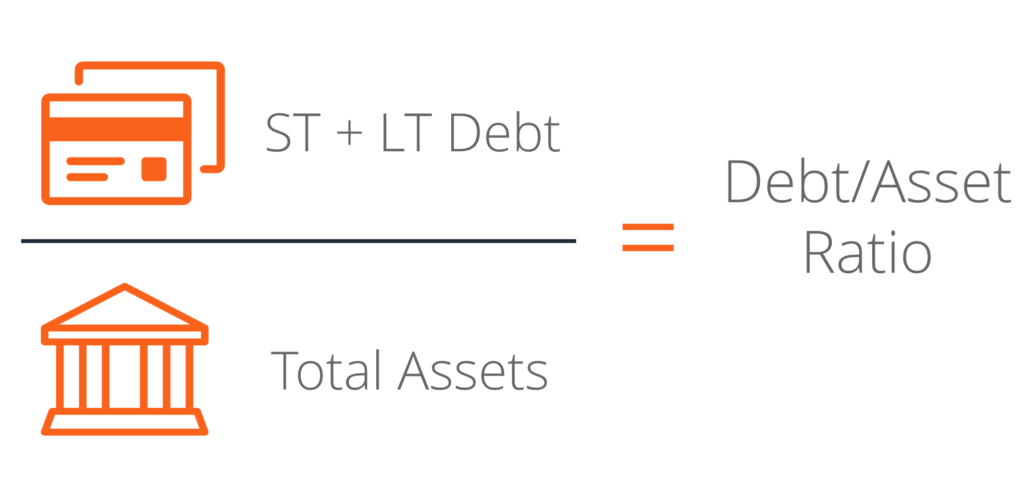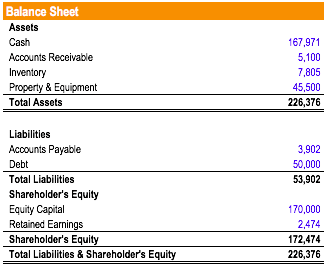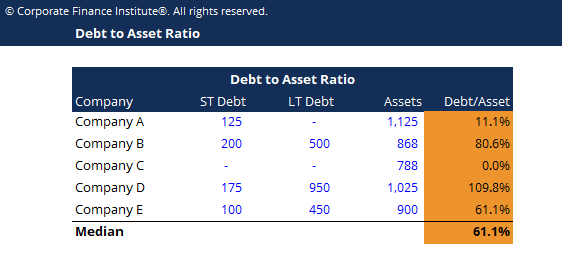# Debt to Asset Ratio

A leverage ratio that indicates the percentage of assets that are being financed with debt

## What is the Debt to Asset Ratio?

The Debt to Asset Ratio, also known as the debt ratio, is a leverage ratio that indicates the percentage of assets that are being financed with debt. The higher the ratio, the greater the degree of leverage and financial risk.

The debt to asset ratio is commonly used by creditors to determine the amount of debt in a company, the ability to repay its debt, and whether additional loans will be extended to the company. On the other hand, investors use the ratio to make sure the company is solvent, is able to meet current and future obligations, and can generate a return on their investment.### Debt to Asset Ratio Formula

The formula for the debt to asset ratio is as follows:

Debt/Asset = (Short-term Debt + Long-term Debt) / Total Assets

Where:

• Total Assets may include all current and non-current assets on the company’s balance sheet, or may only include certain assets such as Property, Plant & Equipment (PP&E), at the analyst’s discretion.

### Example of Debt to Asset Ratio

Consider the balance sheet below:From the balance sheet above, we can determine that the total assets are \$226,365 and that the total debt is \$50,000. Therefore, the debt to asset ratio is calculated as follows:

Debt to Asset Ratio = \$50,000 / \$226,376 = 0.2208 = 22%

Therefore, the figure indicates that 22% of the company’s assets are funded via debt.

### Interpretation of Debt to Asset Ratio

The debt to asset ratio is commonly used by analysts, investors, and creditors to determine the overall risk of a company. Companies with a higher ratio are more leveraged and hence, riskier to invest in and provide loans to. If the ratio steadily increases, it could indicate a default at some point in the future.

• A ratio equal to one (=1) means that the company owns the same amount of liabilities as its assets. It indicates that the company is highly leveraged.
• A ratio greater than one (>1) means the company owns more liabilities than it does assets. It indicates that the company is extremely leveraged and highly risky to invest in or lend to.
• A ratio of less than one (<1) means the company owns more assets than liabilities and can meet its obligations by selling its assets if needed. The lower the debt to asset ratio, the less risky the company.

Let us examine the debt to asset ratio of five hypothetical companies:Company D shows a significantly higher degree of leverage compared to the other companies. Therefore, Company D would see a lower degree of financial flexibility and would face significant default risk if interest rates were to rise. If the economy were to undergo a recession, Company D would more than likely be unable to stay afloat.

On the flip side, if the economy and the companies performed very well, Company D could expect to have the highest equity returns, due to its leverage.

Company C would have the lowest risk and lowest expected return (all else being equal).

### Key Takeaways

The debt to asset ratio is very important in determining the financial risk of a company. A ratio greater than 1 indicates that a significant portion of assets is funded with debt and that the company may be facing default risk. Therefore, the lower the debt to asset ratio, the safer the company. As with any other ratios, the ratio should be evaluated over a period of time to access whether the company’s financial risk is improving or deteriorating.

### Other Resources

CFI is the official provider of the global Financial Modeling & Valuation Analyst (FMVA)™ certification program, designed to help anyone become a world-class financial analyst. To keep advancing your career, the additional resources below will be useful:

• Debt Capacity
• Cost of Debt
• Financial Leverage
• Capital Structure

### Financial Analyst Training

Get world-class financial training with CFI’s online certified financial analyst training program!

Gain the confidence you need to move up the ladder in a high powered corporate finance career path.

Learn financial modeling and valuation in Excel the easy way, with step-by-step training.